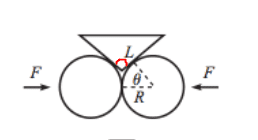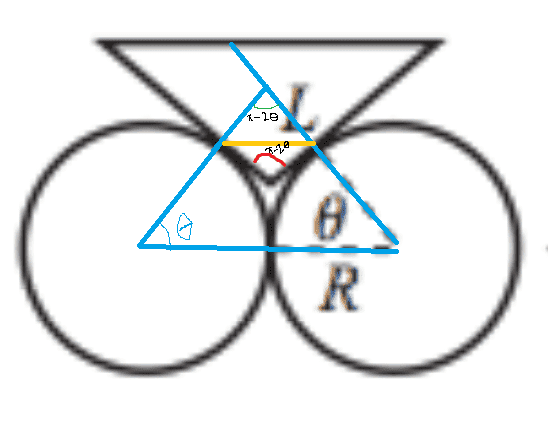# Finding angle of a reflected isosceles triangle

Brown Arrow

## Homework Statement

I am trying to find the following (marked in red) angle, I know that the radii to the points of contact make an angle θ with the horizontal.## Homework Equations

all angles of a triangle add up to 180 degrees.
isosceles triangle, has two equivalent sides

## The Attempt at a SolutionI tried using symmetry and found the part in red is $\pi$ - 2$\theta$
but according to the text, the angle is 2$\theta$. I am confused on why is is 2$\theta$

Homework Helper
The sides of the inverted triangle are tangents to each circle.
The base of your blue triangle is 2R.
The angles on either side of the base must be ##\theta##.

The blue-triangle apex angle must be ##\pi-2\theta## ... which is where you've got up to.

The apex of the blue triangle forms a diamond shape with the inverted triangle.
The bottom of the diamond is the angle you want.
The left-hand angle is the same as the right-hand angle - you actually know what these angles are!

What do the angles in the diamond add up to?
What do the left hand and right hand angles add up to?